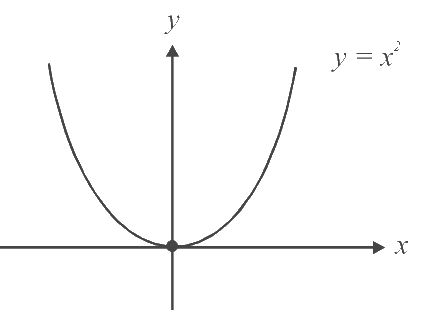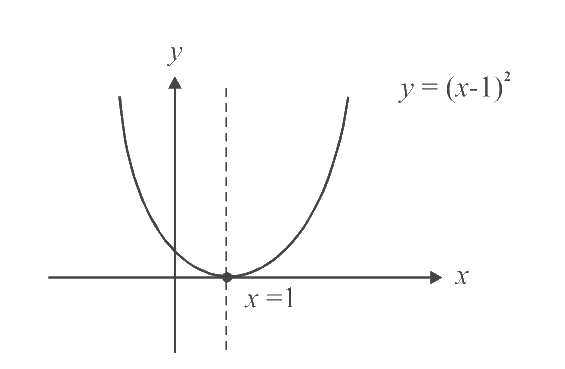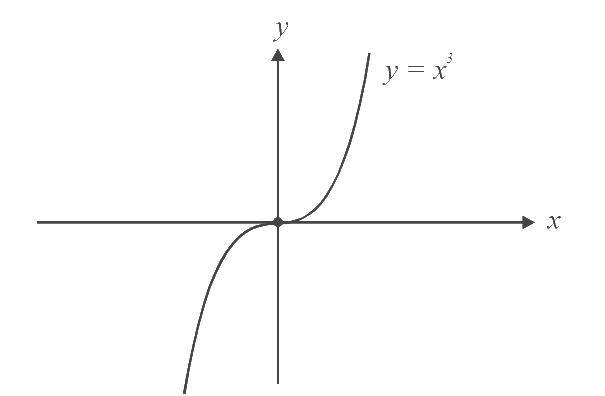# Even and odd Nature of Functions

Go back to  'Functions'

## EVEN / ODD FUNCTIONS

A function is even if $$f(x) = f( - x).$$ This means that the function is the same for $$\text{+ve } x\text{-axis}$$ and $$\text{-ve } x\text{-axis,}$$ or graphically, symmetric about the $$y\text{-axis}$$

For example, $$f(x) = {x^2}$$ is evenA function is even about $$'a'$$ if it is symmetric about the line $$x=a.$$ For example, $$f(x) = {(x - 1)^2}$$ is even about $$x=1.$$For such a function $$f(a - x) = f(a + x)$$

A function is odd if $$f(x)= f(-x),$$ that is, the function on one side of $$x\text{-axis}$$ is sign inverted with respect to the other side or graphically, symmetric about the origin.

For example, $$f(x) = {x^3}$$ is odd.As in the even case, $$f(x)$$ can be odd about $$'a'.$$ For example, $$f(x) = {(x + 2)^3}$$ is odd about $$x=-2.$$

A function can also be neither even nor odd.

For example, $$f(x)={x^2}+{x^3},\text{ as }f(x)\ne f(-x)\text{ and }f(x)\ne- f(-x)$$

Example - 34

Check whether the following functions are even or odd.

\begin {align} {\bf{(a)}} \ \; f(x) = \sin x - \cos x\qquad \qquad {\bf{(b)}} \ \; f(x) = \frac{x}{{{e^x} - 1}} + \frac{x}{2} + 1 \qquad \qquad {\bf{(c)}} \ \; f(x) = \sqrt{{{{(1 - x)}^2}}} + \sqrt{{{{(1 + x)}^2}}}\end {align}

Solution:

(a)  $$f(-x)=\sin(- x)-\cos(-x)=-{\text{sin}}x-{\text{cos}}x$$

We see that  $$f( - x) \ne f(x)$$   and   $$f( - x) \ne - f(x)$$

Hence, this function is neither even nor odd.

(b)  f( - x) =\begin{align} \frac{{ - x}}{{{e^{ - x}} - 1}} - \frac{x}{2} + 1= \frac{{x{e^x}}}{{{e^x} - 1}} - \frac{x}{2} + 1\end{align}

= \begin{align}\frac{{x{e^x} + x}}{{2({e^x} - 1)}} + 1=\frac{{x({e^x} - 1) + 2x}}{{2({e^x} - 1)}} + 1=\frac{x}{{{e^x} - 1}} + \frac{x}{2} + 1 = f(x)\end{align}

Therefore, this function is even.

(c)  $$f( - x) = f(x)$$, which is obvious just by observation.

This function is even.

Example – 35

If the function $$f$$ satisfies $$f(x + y) + f(x - y) = 2f(x).\,f(y)$$  $$\forall x, y \; in\; \mathbb{R}$$, and  f(0) $$\ne$$ 0, prove that $$f(x)$$ is even

Solution:

Substituting $$x=y=0,$$

we get   $$2f(0) = 2f{(0)^2}$$      $$\Rightarrow$$    $$f(0)\,\,(f(0) - 1) = 0$$

Since   $$f(0)$$ $$\ne$$ 0,    we have   $$f(0)$$  = 1

Now substitute $$x=0,$$

to get        $$f(y) + f( - y) = 2f(0)f(y) = 2f(y)$$

$$\Rightarrow$$         $$f(y) = f( - y)$$

$$\Rightarrow$$         $$f$$ is even.

Example – 36

If  $$f(x + y) = f(x).f(y)\forall$$  real  $$x,\,y$$  and  $$f(0) \;\ne\,0,$$  then prove that the function f(x) = \begin{align}\frac{{f(x)}}{{1 + {{\left( {f(x)} \right)}^2}}}\end{align} is an even function.

Solution:

As in the previous question, we first substitute  $$x=y=0$$  to get  $$f(0)=1$$  $$\Rightarrow$$ f(x) =\begin{align} \frac{1}{{f( - x)}}\end{align}

(Note that the approach in such questions is always to try out some sample substitutions that yield significant results).

$Now \qquad\qquad\qquad f( - x) = \frac{{f( - x)}}{{1 + {{\left( {f( - x)} \right)}^2}}} = \frac{{\frac{1}{{f(x)}}}}{{1 + {{\left( {\frac{1}{{f(x)}}} \right)}^2}}} = \frac{{f(x)}}{{1 + {{\left( {f(x)} \right)}^2}}} = f(x).$

Hence, $$f(x)$$ is even.

Functions
grade 11 | Questions Set 1
Functions
Functions
grade 11 | Questions Set 2
Functions